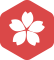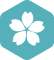### math.random()

Java > Java SE [问题点数：20分，结帖人wwwmmmjjj1234567890]2009年4月 Java大版内专家分月排行榜第一wwwmmmjjj1234...

math.random()函数用法

math.random()函数是取[0,1)之间的随机数，则math.random()*100表示[0,100)之间的随机数，(math.random()*40)*10表示[0,400)之间的随机数，如需取整则int((math.random()*40)*10)这样。

Math.random()的用法

Math.random( ) 的使用 1.介绍： Math.random()是令系统随机选取大于等于 0.0 且小于 1.0 的伪随机 double 值 2.使用方法： 例： int num = (int)(Math.random()*2+1) //以上代码即设置一个随机1到3(取不到...

Random()、Math.random()、Math.round()函数

1.Random()、Math.random()、Math.round()函数的区别;1.Random()、Math.random()、Math.round()函数的区别;1.Random()、Math.random()、Math.round()函数的区别;1）Random()：创建一个新的随机数生成器。此构造方法...

Math.random函数就不像php的rand函数一样可以生成指数范围的数据了，math.random只是生成了一个伪随机数，之后还要经过我们处理才行哦。   今天有又网友问到我 JavaScript 怎么生成指定范围数值随机数。Math....

Java 基础之 Random类和Math.random()方法

Random类 import java.util.Random; public class randomTest { public static void main(String args[]) { Random random=new Random();//以系统当前时间作为随机数生成的种子... System.out.println(random....

Math.random()

Math.random() 作用是返回一个0到1之间的随机数 &lt;script&gt; var x = Math.random(); document.write(x); &lt;/script&gt;

java Math.random()

Math.random()是令系统随机选取大于等于 0.0 且小于 1.0 的伪随机 double 值 公式：Math.random()*(n-m)+m，生成大于等于m小于n的随机数； 例如：定义一个随机1到5(取不到5)的变量 [1,5) int number=(int)(Math....

Math.random()实际应用

Math.random()返回0到1之间的一个伪随机数，可能等于0，但是一定小于1。 Math.random() // 0.7151307314634323 复制代码 任意范围的随机数生成函数如下。 function getRandomArbitrary(min, max) { return Math....

Math.random()的使用

Math.random()是用于随机生成一个［0.0, 1.0) 的伪随机 double 值. 随机生成［a, b)： (int)(Math.random()*(b-a)+a) 随机生成［a, b］ (int)(Math.random()*(b-a+1)+a)

Math.floor( Math.random() )生成随机整数

Math.random():获取0~1随机数 Math.floor() method rounds a number DOWNWARDS to the nearest integer, and returns the result. (小于等于 x，且与 x 最接近的整数。) 其实返回值就是该数的整数位： Math.floor(0....

math.random的取值范围

x=math.random*A; 这里随机取到的值的范围：0

Math.random()生成随机数方法

Math.random()生成随机数方法该方法返回 0 到 1 之间的一个伪随机数，可能等于 0，但是一定小于 1。 Math.random() // 0.7151307314634323 // 返回给定范围内的随机数(上面的方法是随机整数)function ...

JAVA中Math类的random()方法使用

java中Math.random()与java.util.random()的区别

echarts地图从全国进省从省进市

Math.Random()方法的使用

Math.Random()会随机生成[0，1)之间的随机数，一般情况下大家让Math.Random()乘以一个数,例如a。这样我们就可以生成[0，a)之间的随机数([0*a=0,1*a=a)),如果再在前面添加一个int,那么我们就可以产生[0，a)之间的正...

js Math.random() 随机数 1-10之间

java中的int i=(int)(Math.random()*100)+1;怎样理解？

Math.random()返回一个double类型的0.0-1.0之间的数值，包括0，不包括1，这个数值*100，那范围不就是0-100(不包括)了么。强转之后范围就是0-99,+1

java 使用RandomMath.random()去生成10以内的随机数

import java.util.Random; /** * Created by kdoulf on 2017/4/6. */ public class RandomTest { public static void main... //10以内的随机数可以使用两种方法，一个是Random一个是Math.random()*10,Math.

js Math.random()在指定的范围内生成随机数

Math.random()和Math.round()总结

Lua库函数——math.randomseed()、os.time()的使用

random.nextInt()与Math.random()基础用法

1、来源java.util.Random类中的方法；Math 类中的静态方法2、用法产生0－n的伪随机数(伪随机数参看最后注解)：// 两种生成对象方式：带...Integer res = random.nextInt(n);Integer res = (int)(Math.random() * n);3、

Math.random()函数生成n到m间的随机数字

Math.random()函数返回0和1之间的伪随机数，可能为0，但总是小于1，[0,1) 生成n-m，包含n但不包含m的整数： 第一步算出 m-n的值，假设等于w 第二步Math.random()*w 第三步Math.random()*w+n 第四步...

MathRandom获取随机数

Android Math.random()解释

Math.random()：产生一个[0，1)之间的随机数,大于等于0小于1的随机小数，返回值是double类型的。 eg:int value=（int）(Math.random()*x+y);//生成大于等于0*x+y---小于1*x+y之间的随机整数 也就是大于等于y--小于...

java基础_函数Math.random()和Random的使用及区别

Math.random()是令系统随机选取大于等于 0.0 且小于 1.0 的伪随机 double 值，是Java语言常用代码。例如：Number=Math.random()*3+1，设置一个随机1到4的变量。 Random 类有含参数和不含参数的构造；其中不含参的...

echart中国地图，多地图案例

1.多地图案例 2.中国地图

java中RandomMath.random用法 生成int随机数